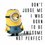# I Don't Get It!

I came across a question which uses Fermat's little theorem:

The Question:
Find a positive integer n such that $7n^{25}$ - 10 is divisible by 83.

The Solution given in the book:
Since 7 x 37 = 259 = 10 mod 83
We have to find a value of n such that $7n^{25}$ = 7 x 37 mod 83
This is equivalent to $n^{25}$ = 37 = $2^{20}$ mod 83
By Fermat's Theorem,
$2^{82k}$ = 1 mod 83 for all k.
So we need to choose n such that $n^{25}$ =$2^{82k+20}$ mod 83
This will be satisfied if k=15
Therefore $n^{25}$ = $2^{1250}$ mod 83
And so n = $2^{50}$
This gives one value.

My problem is that I don't understand the fourth line

That is how 37 = $2^{20}$ mod 83?

So can someone please explain this?Note by Abc Xyz
5 years, 4 months ago

This discussion board is a place to discuss our Daily Challenges and the math and science related to those challenges. Explanations are more than just a solution — they should explain the steps and thinking strategies that you used to obtain the solution. Comments should further the discussion of math and science.

When posting on Brilliant:

• Use the emojis to react to an explanation, whether you're congratulating a job well done , or just really confused .
• Ask specific questions about the challenge or the steps in somebody's explanation. Well-posed questions can add a lot to the discussion, but posting "I don't understand!" doesn't help anyone.
• Try to contribute something new to the discussion, whether it is an extension, generalization or other idea related to the challenge.

MarkdownAppears as
*italics* or _italics_ italics
**bold** or __bold__ bold
- bulleted- list
• bulleted
• list
1. numbered2. list
1. numbered
2. list
Note: you must add a full line of space before and after lists for them to show up correctly
paragraph 1paragraph 2

paragraph 1

paragraph 2

[example link](https://brilliant.org)example link
> This is a quote
This is a quote
    # I indented these lines
# 4 spaces, and now they show
# up as a code block.

print "hello world"
# I indented these lines
# 4 spaces, and now they show
# up as a code block.

print "hello world"
MathAppears as
Remember to wrap math in $$ ... $$ or $ ... $ to ensure proper formatting.
2 \times 3 $2 \times 3$
2^{34} $2^{34}$
a_{i-1} $a_{i-1}$
\frac{2}{3} $\frac{2}{3}$
\sqrt{2} $\sqrt{2}$
\sum_{i=1}^3 $\sum_{i=1}^3$
\sin \theta $\sin \theta$
\boxed{123} $\boxed{123}$

Sort by:

$2^8 \equiv 256 \equiv 249 + 7 \equiv 7$ (mod $83$)
$\Rightarrow 2^{16} \equiv 7^2$ (mod $83$)
$\Rightarrow 2^{20} \equiv 49 \cdot 16 \equiv 784 \equiv 37$(mod $83$)

- 5 years, 4 months ago

Wow !! I didn't think of that. Thanks a LOT Sir !!!

- 5 years, 4 months ago

Note that 2 is a primitive root modulo 83, so that even if the remainder weren't 37, we could find another exponent (but finding the exact exponent is difficult, in general.) instead of 20 here.

- 5 years, 4 months ago